PointsBet World Series Odds
+450
4.5 to 1Dodgers
14.8% implied probability

+600
6 to 1Yankees
11.6% implied probability

+800
8 to 1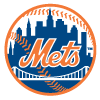Mets
9.0% implied probability

+850
8.5 to 1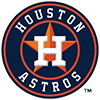Astros
8.6% implied probability

+1000
10 to 1Brewers
7.4% implied probability

+1000
10 to 1Blue Jays
7.4% implied probability

+1500
15 to 1Braves
5.1% implied probability

+1600
16 to 1Padres
4.8% implied probability

+1600
16 to 1White Sox
4.8% implied probability

+2000
20 to 1Giants
3.9% implied probability

+2000
20 to 1Rays
3.9% implied probability

+2000
20 to 1Angels
3.9% implied probability

+2500
25 to 1Twins
3.1% implied probability

+3000
30 to 1Phillies
2.6% implied probability

+3300
33 to 1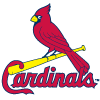Cardinals
2.4% implied probability

+6000
60 to 1Red Sox
1.3% implied probability

+6000
60 to 1Mariners
1.3% implied probability

+8000
80 to 1Marlins
1.0% implied probability

+10000
100 to 1Guardians
0.8% implied probability

+25000
250 to 1Royals
0.3% implied probability

+25000
250 to 1Tigers
0.3% implied probability

+25000
250 to 1Rockies
0.3% implied probability

+25000
250 to 1Rangers
0.3% implied probability

+25000
250 to 1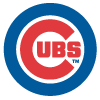Cubs
0.3% implied probability

+50000
500 to 1Diamondbacks
0.2% implied probability

+50000
500 to 1Pirates
0.2% implied probability

+100000
1000 to 1Athletics
0.1% implied probability

+100000
1000 to 1Reds
0.1% implied probability

+100000
1000 to 1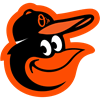Orioles
0.1% implied probability

+100000
1000 to 1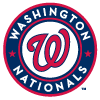Nationals
0.1% implied probability